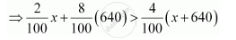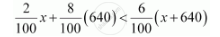CBSE (Arts) Class 11CBSE
Share

# A Solution of 8% Boric Acid is to Be Diluted by Adding a 2% Boric Acid Solution to It. the Resulting Mixture is to Be More than 4% but Less than 6% Boric Acid. If We Have 640 Litres of the 8% Solution, How Many Litres of the 2% Solution Will Have to Be Added? - CBSE (Arts) Class 11 - Mathematics

ConceptGraphical Solution of Linear Inequalities in Two Variables

#### Question

A solution of 8% boric acid is to be diluted by adding a 2% boric acid solution to it. The resulting mixture is to be more than 4% but less than 6% boric acid. If we have 640 litres of the 8% solution, how many litres of the 2% solution will have to be added?

#### Solution

Let x litres of 2% boric acid solution is required to be added.

Then, total mixture = (x + 640) litres

This resulting mixture is to be more than 4% but less than 6% boric acid.

∴2%x + 8% of 640 > 4% of (x + 640)

And, 2% + 8% of 640 < 6% of (x + 640)

2%x + 8% of 640 > 4% of (x + 640)⇒ 2x + 5120 > 4x + 2560

⇒ 5120 – 2560 > 4x – 2x

⇒ 5120 – 2560 > 2x

⇒ 2560 > 2x

⇒ 1280 > x

2% x + 8% of 640 < 6% of (x + 640)⇒ 2x + 5120 < 6x + 3840

⇒ 5120 – 3840 < 6x – 2x

⇒ 1280 < 4x

⇒ 320 < x

∴320 < x < 1280

Thus, the number of litres of 2% of boric acid solution that is to be added will have to be more than 320 litres but less than 1280 litres.

Is there an error in this question or solution?

#### APPEARS IN

NCERT Solution for Mathematics Textbook for Class 11 (2018 to Current)
Chapter 6: Linear Inequalities
Q: 12 | Page no. 132

#### Video TutorialsVIEW ALL 

Solution A Solution of 8% Boric Acid is to Be Diluted by Adding a 2% Boric Acid Solution to It. the Resulting Mixture is to Be More than 4% but Less than 6% Boric Acid. If We Have 640 Litres of the 8% Solution, How Many Litres of the 2% Solution Will Have to Be Added? Concept: Graphical Solution of Linear Inequalities in Two Variables.
S